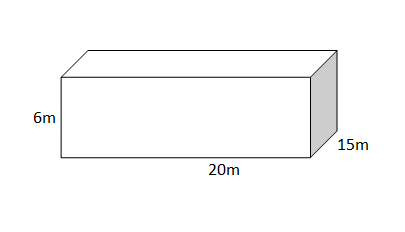QuestionAnswers

# A village having a population of 4000 requires 150 litres of water per head per day. It has a tank measuring $20m\times 15m\times 6m$. For how many days will the water of this tank last?Verified
128.7k+ views
Hint: We have been given the amount of water required by the villagers per head per day and the dimensions of the tank. To find the number of days the water will last we will need to find out two things –total water requirement of the village per day and the volume of the water. We can find the total water required by multiplying the population of the village with per head per day water requirement. After finding out both these things we can find out the number of days the water will last by the dividing the volume of the tank given by $20\times 15\times 6{{m}^{3}}=20\times 15\times 6\times {{10}^{3}}lit$ with the total water requirement and we will have our answer.

Now, we have been given that the population of the village is 4000 and it has a water requirement of $150lit$.
Therefore, the total water requirement of the village per day is given by multiplying the village population with the per head per day water requirement.
\begin{align} & \Rightarrow 4000\times 150lit \\ & \Rightarrow 600000lit \\ \end{align}
Thus, the total water requirement per day is 600000 lit.
Now, we will find the volume of the tank.
We have been given the dimension of the tank as $20m\times 15m\times 6m$. Thus, the tank is in cuboidal shape.We know that the volume of a cuboid is given by $l\times b\times h$ where l is the length, b is the breath and h is the height of the tank.
Therefore, the volume of the tank is given as:
\begin{align} & \Rightarrow 20\times 15\times 6{{m}^{3}} \\ & \Rightarrow 1800{{m}^{3}} \\ \end{align}
Thus, the volume of the tanks is $1800{{m}^{3}}$.
Now, the volume calculated is in cubic metres whereas the total water requirement per day is in litres. So we need to convert cubic metres into litres so that the unit will be the same.
We know that $1{{m}^{3}}=1000lit$
Thus, the volume of the tank in ‘lit’ will come out as:
\begin{align} & \Rightarrow 1800\times 1000lit \\ & \Rightarrow 1800000lit \\ \end{align}
Thus we have a total of 18000000lit in the tank and the total water requirement per day is 600000lit.
So, the number of days water will last will be equal to:
\begin{align} & \Rightarrow \dfrac{1800000}{600000}days \\ & \Rightarrow 3days \\ \end{align}

Thus, the water in the tank will last for 3 days.

Note: Don’t forget to convert the units and make them the same otherwise the answer will not come out to be as the correct one. Here, we have changed cubic metres into litres but we can also change litres into cubic metres. The answer will be the same and correct in both the cases.